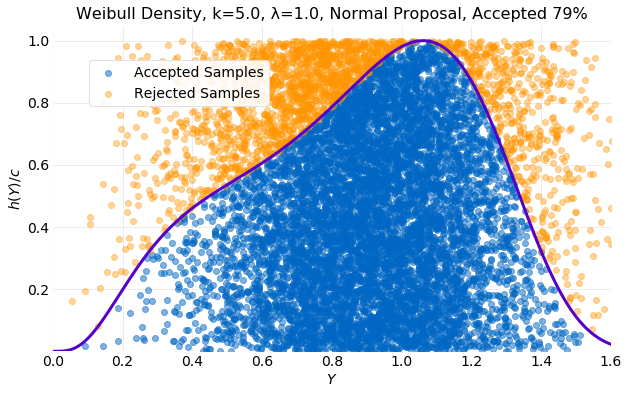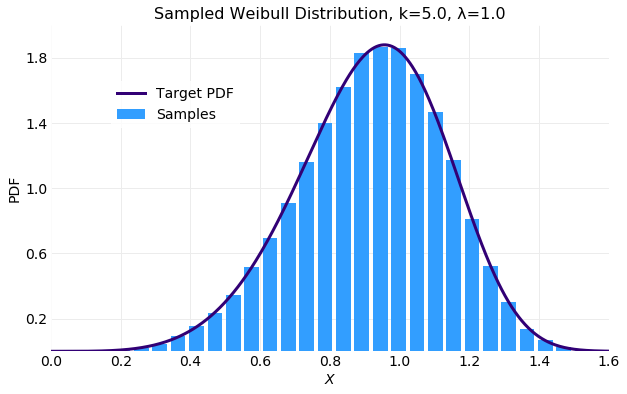• # Rejection SamplingRejection Sampling is a method for obtaining samples for a known target probability distribution using samples from some other proposal distribution. It is a more general method than Inverse CDF Sampling which requires distribution to have an invertible CDF. Inverse CDF Sampling transforms a $\textbf{Uniform}(0,\ 1)$ random variable into a random variable with a desired target distribution using the inverted CDF of the target distribution. While, Rejection Sampling is a method for transformation of random variables from arbitrary proposal distributions into a desired target distribut... read more

• # Inverse CDF SamplingInverse CDF sampling is a method for obtaining samples from both discrete and continuous probability distributions that requires the CDF to be invertible. The method assumes values of the CDF are Uniform random variables on $[0, 1]$. CDF values are generated and used as input into the inverted CDF to obtain samples with the distribution defined by the ... read more